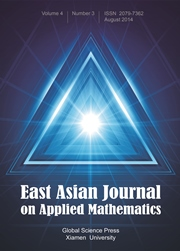Home
Hostname: page-component-544b6db54f-n9d2k Total loading time: 0.162 Render date: 2021-10-17T23:40:35.699Z Has data issue: true Feature Flags: { "shouldUseShareProductTool": true, "shouldUseHypothesis": true, "isUnsiloEnabled": true, "metricsAbstractViews": false, "figures": true, "newCiteModal": false, "newCitedByModal": true, "newEcommerce": true, "newUsageEvents": true }East Asian Journal on Applied Mathematics

# Convergence Analysis for a Three-Level Finite Difference Scheme of a Second Order Nonlinear ODE Blow-Up Problem

Published online by Cambridge University Press:  31 January 2018

*
*Corresponding author. Email address:chcho20@ccu.edu.tw (C.-H. Cho)

## Abstract

We consider the second order nonlinear ordinary differential equation u″ (t) = u1+α (α > 0) with positive initial data u(0) = a0, u′(0) = a1, whose solution becomes unbounded in a finite time T. The finite time T is called the blow-up time. Since finite difference schemes with uniform meshes can not reproduce such a phenomenon well, adaptively-defined grids are applied. Convergence with mesh sizes of certain smallness has been considered before. However, more iterations are required to obtain an approximate blow-up time if smaller meshes are applied. As a consequence, we consider in this paper a finite difference scheme with a rather larger grid size and show the convergence of the numerical solution and the numerical blow-up time. Application to the nonlinear wave equation is also discussed.

## MSC classification

Type
Research Article
Information
East Asian Journal on Applied Mathematics , November 2017 , pp. 679 - 696

## Access options

Get access to the full version of this content by using one of the access options below. (Log in options will check for institutional or personal access. Content may require purchase if you do not have access.)

## References

 Abia, L., López-Marcos, J.C., and J. Martínez, The Euler method in the numerical integration of reaction-diffusion problems with blow-up, Appl. Numer. Math. 38, 287313 (2001).CrossRefGoogle Scholar
 Bizoń, P., Chmaj, T., and Szpak, N., Dynamics near the threshold for blowup in the one-dimensional focusing nonlinear Klein-Gordon equation, J. Math. Phys. 52, 103703–11 (2011).CrossRefGoogle Scholar
 Brandle, C. and Brunner, H., Blow-up in diffusion equations: a survey, J. Comput. Appl. Math. 97, 322 (1998).CrossRefGoogle Scholar
 Chen, Y.-G., Asymptotic behaviours of blowing-up solutions for finite difference analogue of ut = uxx + u 1+α, J. Fac. Sci. Univ. Tokyo 33, 541574 (1986).Google Scholar
 Cho, C.-H., A finite difference scheme for blow-up solutions of nonlinear wave equations, Numer. Math.: Theo. Methods Appl. 3, 475498 (2010).Google Scholar
 Cho, C.-H., On the convergence of numerical blow-up time for a second order nonlinear ordinary equation, Appl. Math. Lett. 24, 4954 (2011).CrossRefGoogle Scholar
 Cho, C.-H., Stability for the finite difference schemes of the linear wave equation with non-uniform time meshes, Numer. Method Partial Differe. Equat. 29, 10311042 (2013).CrossRefGoogle Scholar
 Cho, C.-H., Hamada, S., and Okamoto, H., On the finite differnece approximation for a parabolic blow-up problem, Japan J. Indus. Appl. Math. 24, 131160 (2007).CrossRefGoogle Scholar
 Friedman, A. and McLeod, B., Blow-up of positive solutions of semilinear heat equations, Indiana Univ. Math. J. 34, 425447 (1985).CrossRefGoogle Scholar
 Glassey, R. T., Finite-time blow-up for solutions of nonlinear wave equations, Math. Z. 177, 323340 (1981).CrossRefGoogle Scholar
 Groisman, P., Totally discrete explicit and semi-implicit Euler methods for a blow-up problem in several space dimensions, Computing 76, 325352 (2006).CrossRefGoogle Scholar
 John, F., Blow-up of solutions of nonlinear wave equations in three space dimensions, Manuscripta Math. 28, 235268 (1979).CrossRefGoogle Scholar
 Kato, T., Blow-up of solutions of some nonlinear hyperbolic equations, Comm. Pure Appl. Math. 32, 501505 (1980).CrossRefGoogle Scholar
 Levine, H., Instability and nonexistence of global solutions to nonlinear wave equations of the form Putt = −Au + F(u), Trans. Amer. Math. Soc. 192, 121 (1974).Google Scholar
 Killip, R., Stovall, B. and Visan, M., Blowup behavior for the nonlinear Klein-Gordon equation, Math. Ann. 358, 289350 (2014).CrossRefGoogle Scholar
 Matus, Piotr P., Mazhukin, Vladimir I., and Mozolevsky, Igor E., Stability of finite difference schemes on non-uniform spatial-time-grids, NAA 2000, LNCS 1988, 568577 (2001).Google Scholar
 Matus, P. and Zyuzina, E., Three-level difference schemes on non-uniform in time grids, Comput. Methods Appl. Math. 1, 265284 (2001).CrossRefGoogle Scholar
 Nakagawa, T., Blowing up of a finite difference solution to ut = uxx +u 2, Appl. Math. Optim. 2, 337350 (1976).CrossRefGoogle Scholar
 Saito, N. and Sasaki, T., Blow-up of finite-difference solutions to nonlinear wave equations, J. Math. Sci. Univ. Tokyo 23, 349380 (2016).Google Scholar
 Samarskii, A. A., Vabishchevich, P. N., Makarevich, E. L., and Matus, P. P., Stability of three-layer difference schemes on time-nonuniform grids, Dokl. Russ. Acad. Nauk 376, 738741 (2001).Google Scholar

# Send article to Kindle

Note you can select to send to either the @free.kindle.com or @kindle.com variations. ‘@free.kindle.com’ emails are free but can only be sent to your device when it is connected to wi-fi. ‘@kindle.com’ emails can be delivered even when you are not connected to wi-fi, but note that service fees apply.

Find out more about the Kindle Personal Document Service.

Convergence Analysis for a Three-Level Finite Difference Scheme of a Second Order Nonlinear ODE Blow-Up Problem
Available formats
×

# Send article to Dropbox

To send this article to your Dropbox account, please select one or more formats and confirm that you agree to abide by our usage policies. If this is the first time you use this feature, you will be asked to authorise Cambridge Core to connect with your <service> account. Find out more about sending content to Dropbox.

Convergence Analysis for a Three-Level Finite Difference Scheme of a Second Order Nonlinear ODE Blow-Up Problem
Available formats
×

# Send article to Google Drive

To send this article to your Google Drive account, please select one or more formats and confirm that you agree to abide by our usage policies. If this is the first time you use this feature, you will be asked to authorise Cambridge Core to connect with your <service> account. Find out more about sending content to Google Drive.

Convergence Analysis for a Three-Level Finite Difference Scheme of a Second Order Nonlinear ODE Blow-Up Problem
Available formats
×
×